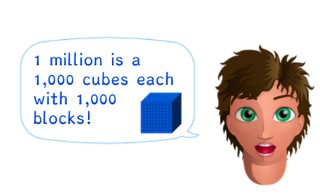Introduction to millions

# Introduction to millions

Introduce students to millions.

No account needed.8,000 schools use Gynzy92,000 teachers use Gynzy1,600,000 students use Gynzy

## General

Students are introduced to millions. They are shown how to write millions in two ways and where millions are placed in the place value chart.

## Standards

CCSS.Math.Content.5.NBT.A.1

## Learning objective

Students will be able to recognize, compare, organize, and write down millions.

## Introduction

Start by saying sets of numbers out loud. You add a zero to the numbers consecutively from ones to ten thousands numbers. Ask students to say the numbers out loud. Then drag the ten thousands number and hundred thousands numbers to the correct boxes.

## Instruction

Check that students have been introduced to millions by asking the following questions:
- How many zeroes does 8 million have?
- How do you write 2.3 million fully?
- How do you write 0.7 million fully?
- Which is more, 3.4 million or 710,000?
- How many more do you need to add to 900,000 to reach 1 million?

## Quiz

Students are given exercises in which they are asked to place given numbers in order from smallest to largest, to write given millions numbers in abbreviated forms fully, as well as determining how many more are needed to reach a million.

## Closing

Discuss the importance of knowing millions and both notations of writing millions. Discuss that you can come across millions numbers in the newspaper. Read the given example on the interactive whiteboard. Then ask students to match the full and abbreviated notations of the numbers. They then must place the given numbers in order from least to greatest. The first number has already been given. Ask students to explain their strategy. To close the lesson, ask students to look up a city that has more than 1 million inhabitants. Then have them write the number of inhabitants as a full and abbreviated number, London is given as an example.

## Teaching tips

Emphasize the fact that 8,100,000 is the same as 8.1 million. The only difference is notation. Have students who have difficulty with these larger numbers first practice with 1 decimal place number, before moving to 2 or 3 decimal places. Students who are ready for a challenge can be given more complex numbers like 8,104,387.

### The online teaching platform for interactive whiteboards and displays in schools

• Save time building lessons

• Manage the classroom more efficiently

• Increase student engagement